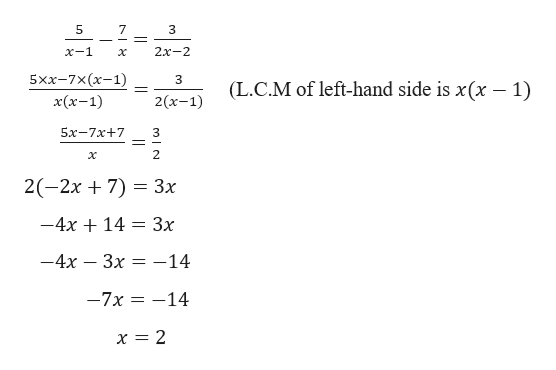# How do I find the least common denominatior of 3 fractions that have the term x? For example, when solving for 5/(x-1) - 7/x = 3/(2x-2), I see that the common denominator for these denominators is 2x(x-1). Why did not all the denominators get multiplied? How do I know what common denominator to use?

Question

How do I find the least common denominatior of 3 fractions that have the term x? For example, when solving for 5/(x-1) - 7/x = 3/(2x-2), I see that the common denominator for these denominators is 2x(x-1). Why did not all the denominators get multiplied? How do I know what common denominator to use?

check_circle

Step 1

Given,

...help_outlineImage Transcriptionclose5 7 2х-2 x-1 5xx-7x(х-1) 3 (L.C.M of left-hand side is x(x -1) x(х-1) 2 (х-1) 5х-7х+7 2 2(-2x 7) 3x -4x 14 3x — 4х — Зх -14 -7x-14 x = 2 LO fullscreen

### Want to see the full answer?

See Solution

#### Want to see this answer and more?

Solutions are written by subject experts who are available 24/7. Questions are typically answered within 1 hour.*

See Solution
*Response times may vary by subject and question.
Tagged in

### Equations and In-equations